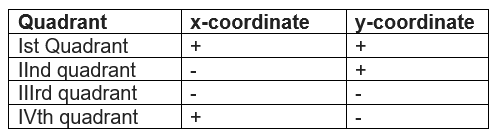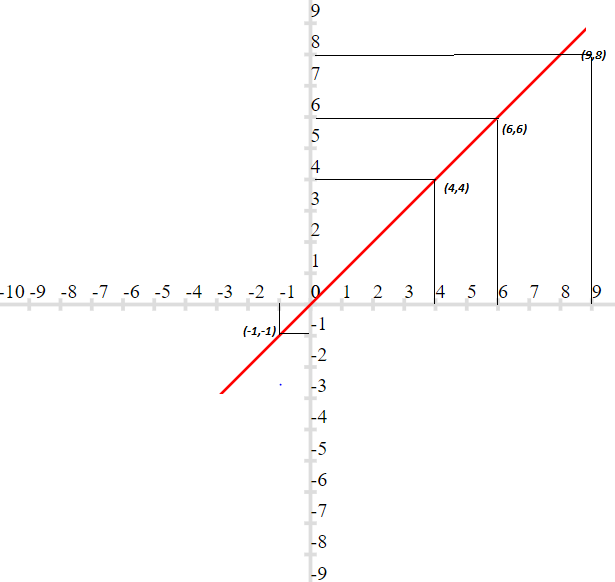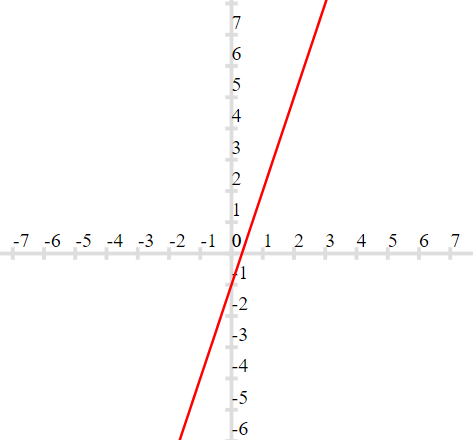# coordinate geometry class 9 extra questions

Given below are the coordinate geometry class 9 extra questions and Class 9 Maths Important Questions for Coordinate Geometry
(a) Concepts questions
(b) Calculation problems
(c) Multiple choice questions
(e) Fill in the blank's
Hope you like this and share with your friends

## coordinate geometry class 9 extra questions

Question 1
State the quadrant for each of these points in Cartesian plane
1. (2,3)
2. (3,-9)
3. (-9,-8)
4. (1,1)
5. (-2,7)
6. (2,0)
7. (0,3)
Solution
We know the quadrant signs are given belowSo we can easily find the quadrant for each of these points

Question 2
Plot the following points in the Cartesian plane
(a) (9, 8)
(b) (-1,-1)
(c) (6, 6)
(d) (4, 4)
Also find which of these three lie are collinear
SolutionQuestion 3
True or False statement (a) x –coordinate is positive in Ist and  IIIrd quadrants
(b) The (0,0) is the coordinate of origin
(c) The point ( 0,2) lies on y axis
(d) The ordinate of the point Q ( 2,3) is 2
(e) Abscissa of all points on y axis is zero
(f) The points P ( 2,3) and Q ( -3,2) lie in the same quadrant
Solution
1. False ,x-axis is positive in I and IV quadrant
2. True
3. True
4. false
5. True
6. False

Question 4
Fill in the blanks (a) The point at which the two coordinate axes meet is called the _____
(b) The point (-10,-11) lies in ____quadrant
(c) The perpendicular distance of the point (11, -12) from the x-axis is _____
(d) The ordinate of the point Q ( 2,3) is _____
(e) (x, y) = (y, x) is valid if _____
(f) distance between the points (2, 0) and (-2, 0) is _____
Solution
1. Origin
2. Third
3. 12
4. 2
5. x=y
6. 4

## coordinate geometry class 9 important questions

Multiple choice Questions Question 4
The perpendicular distance of the point X ( 5,6) from X axis is
(a) 5
(b) 6
(c) 4
(d) 1
Solution
(b)

Question 5
The perpendicular distance of the point X ( 2,3) from Y axis is?
(a)2
(b) 3
(c) 5
(d) None of these
Solution
(a)

Question 6
The points ( other than origin ) whose abscissa and ordinates are same will lie in
(d) none of these
Solution
(a)

Question 7
The positive abscissa lies in which quadrants
(a) I
(b) II
(c) III
(d) IV
Solution
(a), (d)

Question 8
Ordinate of all the points on x-axis is
(a) 0
(b) 1
(c) 2
(d) Any number
Solution
(a)

Question 9
The perpendicular distance of the point P (78, 90) from the y-axis is (A) 90 (B) 78 (C) 80 (D) None of these Solution
(b)

Points A (0, 4), B (0, 0) and C (4, 0) are three vertices of a square ABCD. Plot these points on a graph paper and hence find the coordinates of the vertex D
Solution
Point D is (4,4)

Question 11
Draw the graph for the linear equation
y=3x-1
Solution## Summary

This coordinate geometry class 9 extra questions is prepared keeping in mind the latest syllabus of CBSE . This has been designed in a way to improve the academic performance of the students. If you find mistakes , please do provide the feedback on the mail. You can download this test as pdf also as below